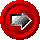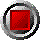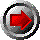Hull-White Model IntroductionHull-White Model Introduction

The Hull-White model is a single-factor, no-arbitrage yield curve model in which the short-term rate of interest is the random factor or state variable (see the Hull text reference). By no-arbitrage, it is meant that the model parameters are consistent with the bond prices implied in the zero coupon yield curve. In addition, yield curve models ensure consistency with the fact that, in the absence of default risk, the price of a bond must pull towards par as it approaches maturity.

The model assumes that the short-term rate is normally distributed and subject to mean reversion. The mean reversion parameter ensures consistency with the empirical observation that long rates are less volatile than short rates. In the special case where the mean reversion parameter is set equal to zero, the Hull-White model reduces to the earlier Ho and Lee model. The use of the normal distribution affords a good deal of analytic tractability, thereby resulting in very fast computation times relative to competing no-arbitrage yield curve models.

The stochastic differential equation describing the form of the Hull-White interest rate model implemented in the software is:where

 dr is the change in the short-term interest rate over a small interval r is the short-term interest rate q(t) is a function of time determining the average direction in which r moves, chosen such that movements in r are consistent with today's zero coupon yield curve a is the mean reversion rate, governing the relationship between short an long rate volatilities dt is a small change in time s is the annual standard deviation of the short rate dz is a Wiener process (a drawing from a standard normal stochastic process)

In practice, the Hull-White model is calibrated by choosing the mean reversion rate and short rate standard deviation in such a way so that they are consistent with option prices observed in the marketplace. Empirical values for the "a" parameter (mean reversion rate) are on the order of 0.0 to 0.1 in North America, while the "s" parameter (short rate standard deviation) tends to be between 0.01 and 0.03. One can use Excel's built-in "Solver" add-in to calibrate these volatility parameters, choosing them such that the mean squared error between the Hull-White model prices and market prices of options are minimized. Sample spreadsheets are available to demonstrate this technique.

The Hull-White Yield Curve Model and Risk Management

In yield curve models such as the Hull-White model, the price of a derivative security is a function of the entire yield curve, rather than a single price as in simpler models like Black-76. The yield curve environment is defined in the Hull-White model by three inputs: a) the zero curve, b) the standard deviation of the short rate, and c) the mean reversion rate [the latter two parameters combine to create the effective volatility].

Single factor models assume that yields for all maturities along the zero curve are instantaneously perfectly correlated. That is, of course, a simplification of reality. As a consequence, it has become accepted practice to "hedge against the model" by computing deltas at various points along the zero curve, thereby gaining insights into the effects of yield curve twists.

Many of the functions in the Hull-White category are designed to facilitate pricing and risk management through the use of bucket shifts of the zero curve. The user can define arbitrary zero curve buckets which are defined by three arguments: Bucket_Start date, Bucket_End date, and Bucket_Shift. These are the last three arguments of many functions in the category. To price an instrument without a bucket shift, set these last three arguments to zero.

Zero curve buckets are designed to include the start date up to, but not including, the end date. It is common to define narrow buckets at the short end of the zero curve, say at monthly intervals, followed by coarser buckets, say at 6-month or yearly intervals.

To solve for the price sensitivity to a shift in the zero curve, price the security with the bucket shift and subtract the price without the shift.

Sensitivity of prices to parallel shifts of the zero curve can easily be effected through use of the OAS (option-adjusted spread) argument. As defined here, the OAS is a parallel shift in the continuously compounded compounded zero curve. Using this concept, it is straightforward to create a measure much like the value of a basis point for a bond by calling on a function twice – once with a positive OAS of 1 basis point, and again with a negative OAS of 1 basis point. The average absolute price change is the zero-coupon based value of a basis point.

For securities with fixed or linear payoffs (such as bonds or swaps), the sum of the bucket deltas across the zero curve is equal to an equivalent size OAS shift. This equivalence will not hold for options and bonds or swaps with embedded options, due to option convexity.

The OAS argument can also be used to ensure consistency between the observed spot price of a bond, and the zero curve. If the market price of a bond differs from the price obtained using an OAS of zero (perhaps by a few cents or more), the OAS term can be adjusted in order to fit the market price of the bond. Then, bond option values obtained using this "fudge factor" OAS are more realistic, since the yield curve model is not mispricing the bond (errors in pricing the bond would be magnified when pricing options on the bond, since options are leveraged instruments).

© 1995-98 Leap of Faith Research Inc.